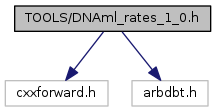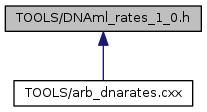ARB
DNAml_rates_1_0.h File Reference
`#include <cxxforward.h>`
`#include <arbdbt.h>`
Include dependency graph for DNAml_rates_1_0.h:This graph shows which files directly or indirectly include this file:Go to the source code of this file.

struct  xarray

struct  node

struct  tree

struct  drawdata

## Macros

#define Debug   1

#define maxsp   10000

#define maxsites   8000

#define maxpatterns   8000

#define maxcategories   35

#define nmlngth   10

#define KI_MAX   256.0

#define RATE_STEP   sqrt(sqrt(2.0))

#define MAX_ERROR   0.01

#define MIN_INFO   4

#define UNDEF_CATEGORY   1

#define zmin   1.0E-15

#define zmax   (1.0 - 1.0E-6)

#define unlikely   -1.0E300

#define decimal_point   '.'

#define LOG(x)   (((x)> 0) ? log(x) : hang("log domain error"))

#define nint(x)   ((int) ((x)>0 ? ((x)+0.5) : ((x)-0.5)))

#define aint(x)   ((double) ((int) (x)))

## Typedefs

typedef double xtype

typedef nodenodeptr

## Variables

const int MAXNODES = 2*maxsp+1

## Macro Definition Documentation

 #define Debug   1

Definition at line 15 of file DNAml_rates_1_0.h.

 #define maxsp   10000

Definition at line 19 of file DNAml_rates_1_0.h.

Referenced by getnums().

 #define maxsites   8000

Definition at line 20 of file DNAml_rates_1_0.h.

Referenced by getnums(), summarize(), and writeToArb().

 #define maxpatterns   8000

Definition at line 21 of file DNAml_rates_1_0.h.

Referenced by makeweights().

 #define maxcategories   35

Definition at line 22 of file DNAml_rates_1_0.h.

Referenced by getoptions(), summarize(), and writeToArb().

 #define nmlngth   10

Definition at line 23 of file DNAml_rates_1_0.h.

Referenced by findTipName(), getdata(), and inputweights().

 #define KI_MAX   256.0

Definition at line 24 of file DNAml_rates_1_0.h.

Referenced by makeUserRates(), and writeToArb().

 #define RATE_STEP   sqrt(sqrt(2.0))

Definition at line 25 of file DNAml_rates_1_0.h.

Referenced by makeUserRates().

 #define MAX_ERROR   0.01

Definition at line 26 of file DNAml_rates_1_0.h.

Referenced by makeUserRates().

 #define MIN_INFO   4

Definition at line 27 of file DNAml_rates_1_0.h.

Referenced by getdata(), and getoptions().

 #define UNDEF_CATEGORY   1

Definition at line 28 of file DNAml_rates_1_0.h.

 #define zmin   1.0E-15

Definition at line 29 of file DNAml_rates_1_0.h.

Referenced by dli_dki(), evaluate(), and newview().

 #define zmax   (1.0 - 1.0E-6)

Definition at line 30 of file DNAml_rates_1_0.h.

 #define unlikely   -1.0E300

Definition at line 31 of file DNAml_rates_1_0.h.

Referenced by findSiteRates(), and setuptree().

 #define decimal_point   '.'

Definition at line 32 of file DNAml_rates_1_0.h.

 #define LOG ( x ) (((x)> 0) ? log(x) : hang("log domain error"))

Definition at line 38 of file DNAml_rates_1_0.h.

 #define nint ( x ) ((int) ((x)>0 ? ((x)+0.5) : ((x)-0.5)))

Definition at line 39 of file DNAml_rates_1_0.h.

Referenced by categorize(), and gb_create_dictionaries().

 #define aint ( x ) ((double) ((int) (x)))

Definition at line 40 of file DNAml_rates_1_0.h.

## Typedef Documentation

 typedef double xtype

Definition at line 43 of file DNAml_rates_1_0.h.

 typedef node* nodeptr

Definition at line 44 of file DNAml_rates_1_0.h.

## Variable Documentation

 const int MAXNODES = 2*maxsp+1

Definition at line 50 of file DNAml_rates_1_0.h.

Referenced by ARB_main().